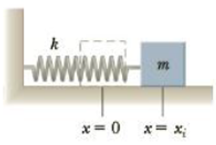Chapter 13, Problem 8P

Chapter
Section
Textbook Problem

A block of mass m = 2.00 kg is attached to a spring of force constant k = 5.00 × 102 N/m that lies on a horizontal frictionless surface as shown in Figure P13.8. The block is pulled to a position xi = 5.00 cm to the right of equilibrium and released from rest. Find (a) the work required to stretch the spring and (b) the speed the block has as it passes through equilibrium.Figure P13.8

a)

To determine
The work required to stretch the string.

Explanation

Given info: The mass of the block is 2.00 kg. The force constant of the spring k is 5.00×102Nm-1 . The displacement of the block from the equilibrium position is 5 cm.

Explanation:

The work done by a spring W   when the displacement from the equilibrium position is x and the spring constant is k is,

W=12kx2

Substitute 5

b)

To determine
The speed of the block when it passes through the equilibrium position.

Still sussing out bartleby?

Check out a sample textbook solution.

See a sample solution

The Solution to Your Study Problems

Bartleby provides explanations to thousands of textbook problems written by our experts, many with advanced degrees!

Get Started